# Learning Properties Of Triangle

In this quiz, different questions regarding the angles and sum of angles of a triangle are asked which also involves the properties of a triangle. Different types of multiple-choice questions and fill-in-the-blanks are asked in the quiz to make the learning more effective.

Start Quiz

Is the given angle acute, obtuse, or right-angled?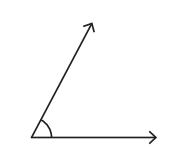Acute angle

Obtuse angle

Right angle

Is the given angle acute, obtuse, or right-angled?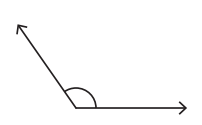Acute angle

Obtuse angle

Right angle

Is the given angle acute, obtuse, or right-angled?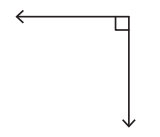Acute angle

Obtuse angle

Right angle

Is the given angle acute, obtuse, or right-angled?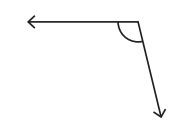Acute angle

Obtuse angle

Right angle

A triangle has side lengths of 5 meters, 6 meters, and 8 meters. What kind of triangle is it?

Equilateral

Isosceles but not equilateral

Scalene

A triangle has side lengths of 10 meters, 6 meters, and 10 meters. What kind of triangle is it?

Equilateral

Isosceles but not equilateral

Scalene

Find the value of b for the given angles of the triangle.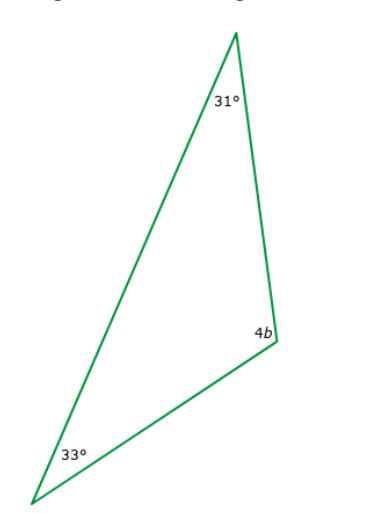b= ##29##

29

Find the value of c for the given angles of the triangle.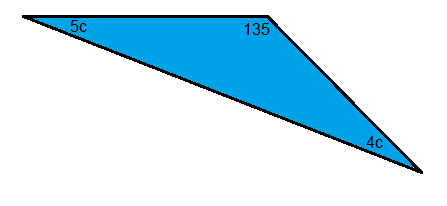c= ##5##

5

Find the value of b for the given angles of the triangle.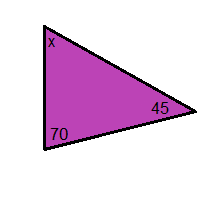x= ##65##

65

Find the value of x for the given angles of the right angle triangle.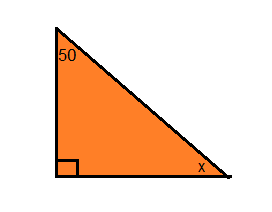b= ##40##

40
Quiz/Test Summary
Title: Learning Properties Of Triangle
Questions: 10
Contributed by: